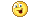#www.vustudents.ning.comkindly ,

all fellows.\

plzzzzzzzzz.

Allah bhla kray sab ka.Views: 750

### Replies to This Discussion

The regular expressions a*|b* and (a|b)* describe the _____set of strings.

Same

Different

Onto

A canonical collection of sets of items for an augmented grammar, C is constructed as -----

For each set / in C and each grammar symbol X where goto (C, X) is empty and not in C add the set goto (C, X) to C.

The first set in C is the closure of {[S' --> .S]}, where S’ is starting symbol of original grammar and S is the starting non-terminal of augmented grammar.

The first set in C is the closure of {[S' --> .S]}, where S is starting symbol of original grammar and S' is the starting non-terminal of original grammar.

S --> a | B B --> Bb | E The non-terminal _______ is left recursive.

------ is a top down parser.

Consider the following grammar, S --> aTUe T --> Tbc/b U --> d And suppose that string “abbcde” can be parsed bottom-up by the following reduction steps: (i) aTbcde (ii) aTde (iii) aTUe (iv) S So, what can be a handle from the following?

The (a) in (aTUe)

The (a) in (aTUe)

The (d) in (aTUe)

.any one has attempted?

who will  help to solve this quiz?In an attribute grammar each production rule(N--> a) has a corresponding attribute evaluation rule that describes how to compute the values of the _______attributes of each particular node N in the AST.

Select correct option:

1. Synthesized
2. Complete
3. Free
4. Bounded

Consider the following grammar, S --> aTUe T --> Tbc/b U --> d And suppose that string “abbcde” can be parsed bottom-up by the following reduction steps: (i) aTbcde (ii) aTde (iii) aTUe (iv) S So, what can be a handle from the following?

Select correct option:

1. The whole string, (aTUe)
2. The whole string, (aTbcde)
3. The whole string, (aTde)
4. None of the given

When constructing an LR(1) parser we record for each item exactly in which context it appears, which resolves many conflicts present in _______parsers based on FOLLOW sets.

Select correct option:

1. SLR(1)
2. LRS(1)
3. RLS(1)
4. None of the given

The ______translation statements can be conveniently specified in YACC

Select correct option:

1. Syntax-directed
2. Image-directed
3. Sign-directed
4. None of the given.

We use ----- to mark the bottom of the stack and also the right end of the input when considering the Stack implementation of Shift-Reduce Parsing.

Select correct option:

1. Epsilon
2. #
3. \$
4. None of the given

Grammars with LL(1) conflicts can be made LL(1) by applying left-factoring, substitution, and left-recursion removal. Left-factoring takes care of ________conflicts.

Select correct option:

1. FIRST/FIRST
2. FIRST/SECOND
3. SECOND/FIRST
4. None of the given

When generating a lexical analyzer from a token description, the item sets (states) are constructed by two types of “moves”: character moves and ____ moves.

Select correct option:

1. E (empty string)
2. #
3. @
4. none of given

Bottom-up parsers handle a _________________ class of grammars.

Select correct option:

1. large
2. small
3. medium
4. none of the given

Let a grammar G = (Vn, Vt, P, S) is modified by adding a unit production S’--> S to the grammar and now starting non-terminals becomes S’ and grammar becomes G’ = (Vn U {S’}, Vt, PU{S’ --> S}, S’). The Grammar G’ is called the -----------

Select correct option:

1. Augmented Grammar
2. Lesser Grammar
3. Anonymous Grammar
4. none of given

The LR(1) items are used as the states of a finite automaton (FA) that maintains information about the parsing stack and progress of a shift-reduce parser.

Select correct option:

1. true
2. false

Backpatching to translate flow-of-control statements in ____ pass.

Select correct option:

1. one
2. two
3. three
4. all of the given

Consider the following grammar, S --> aTUe T --> Tbc/b U --> d And suppose that string “abbcde” can be parsed bottom-up by the following reduction steps: (i) aTbcde (ii) aTde (iii) aTUe (iv) S So what can be a handle from the following?

Select correct option:

1. The second (b) in (abbcde)
2. The first (b) in (abbcde)
3. The substring (cd) in (abbcde)
4. None of the given